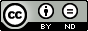### Application of Shooting Type Differential Transform Algorithm for Nonlinear Differential Equations with one Boundary Conditions at Infinity

T. R. Sivakumar, Sudha George

#### Abstract

In this paper, a novel variant of Differential Transform Method (DTM), Shooting Type Differential Transform Algorithm (STDTA) is applied to solve some boundary value problems with one of the boundary conditions at infinity. The analytical solution obtained by using this method converges rapidly and highly effective in terms of accuracy and very close to the exact solution. STDTA is an efficient method for solving these types of problems.

#### Keywords

Nonlinear Differential equations, analytical solutions, STDTA, Pade Approximants, Boundary conditions at infinity

PDF

#### References

. T.R. Sivakumar, Some Variants of Adomian’s Decomposition Method and Their Applications, Ph.D. Thesis, IIT, Madras, 1990.

.T. R. Sivakumar, S. Baiju, Shooting Type Laplace-Adomian Decomposition Algorithm for nonlinear differential equations with boundary conditions at Infinity, Appl. Math. Lett. 24 (2011) 1702-170.

. A. Arikoglu, I. Ozkol, Solution of difference equations by using differential transform method, Appl. Math. and Comput. 174 (2006) 1216-1228.

. P. Darania and A. Ebadian, A method for the numerical solution of the integro-differential equations, Appl. Math. and Comp. 188 (2007) 657-668.

. T. R. Sivakumar, Sudha George, Application of Differential Transform Method to Nonlinear Boundary value Problems, in Proceedings of ICNumACA’ 18, Thiruvananthapuram, 212-219.

. A. Kurnaz, G. Oturanc and M. E. Kiris, n-Dimensional differential transformation method for solving PDES, Int. J. Comput. Math. 82 (2005) 369-380.

. F. Ayas, On the two-dimensional differential transform method, Appl. Math and Comput. 143 (2003) 361-374.

. A. Kurnaz and G. Oturanc, The differential transform approximation for the system of ordinary differential equations, Int. J. Comput. Math. 82 (2005) 709-719.

. H. A. Peker, O. Karaoglu and G. Oturanc, The differential transformation method and Pade approximant for a form of Blasius Equation, Math. and Comput. Appl. 16(2) (2011) 507-513.

. M. Turkyilmazoglu, Some issues on HPM and HAM methods: A convergence scheme. Math. and Comput. Modell. 53 (2011) 1929-1936.

. M. Thiagarajan, K. Senthilkumar, DTM-Pade Approximants for MHD Flow with Suction/Blowing, J. of Appl. Fluid Mechanics, 6 (4) (2013) 537-543.

DOI: https://doi.org/10.24203/ajas.v7i6.6049

### Refbacks

• There are currently no refbacks.